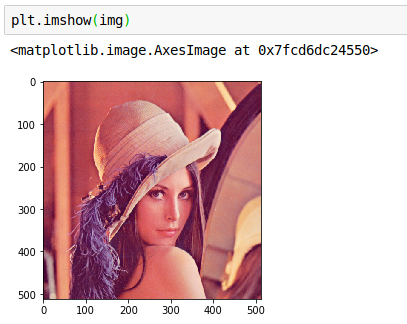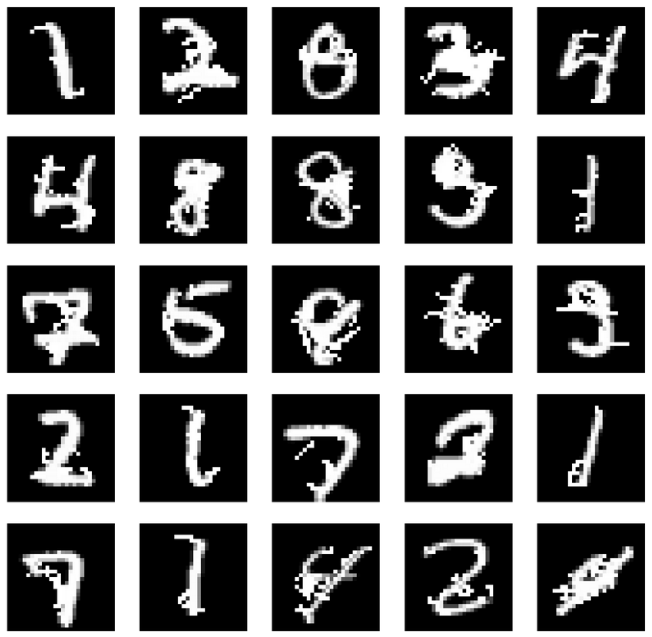### Python图像处理基本操作

• OpenCV（Open Source Computer Vision Library）
• PIL（Python Imaging Library）
• skimage（scikit-image）

*如下代码的实验环境为Jupyter Notebook.

## 1. OpenCV

OpenCV是图像处理中最强大的一个库，它的源代码是由C\C++写成的，所以原版的OpenCV可以与C、C++无缝结合。Python版的OpenCV主要依赖于cv2这个包来实现。

``import cv2import numpy as np #读入图片：默认彩色图，cv2.IMREAD_GRAYSCALE灰度图，cv2.IMREAD_UNCHANGED包含alpha通道img = cv2.imread('Lena.png')print(img.shape)print(type(img))``

```(512, 512, 3)
<class 'numpy.ndarray'>
```

### 1.2  imshow()

``cv2.imshow('Lena',img)cv2.waitKey(0)cv2.destroyAllWindows()``

### 1.3  imwrite()

``cv2.imwrite('lena.jpg',img)``

## 2. PIL

Python里面自带一个PIL（python images library), 但这个库现在已经停止更新了，所以使用Pillow, 它是由PIL发展而来的。

### 1.1  open()

``from PIL import Imageimport numpy as np img = Image.open('Lena.png')print(img.size)print(img.mode)print(type(img))``

```(512, 512)
RGB
<class 'PIL.PngImagePlugin.PngImageFile'>
```

### 1.2 show()

``img.show()``

``import matplotlib.pyplot as plt%matplotlib inline plt.imshow(img)``

inline一句的作用是在Jupyter Notebook的网页中直接绘图，即不会单独弹出绘图窗口，上述代码的执行结果如下：``plt.axis('off')plt.imshow(img)``

``fig=plt.figure(figsize=(28, 28))columns = 5rows = 5for i in range(1, columns*rows +1):    img = test_only_success_adv[650+i].reshape(28,28)+0.5    fig.add_subplot(rows, columns, i)    plt.imshow(img, cmap='gray')    plt.axis('off')``### 1.3  save()

``img.save('lena2.jpg')``

``im = Image.fromarray(np.uint8(data_jsma_0*255))im.save("000.png")``

## 3. skimage

``from skimage import ioimport numpy as np img = io.imread('Lena.png')print(img.shape) # numpy矩阵，(h,w,c) print(type(img))``

```(512, 512, 3)
<class 'numpy.ndarray'>
```

### 1.2  img_as_ubyte()

``print(np.max(adv_test_cw0))print(np.min(adv_test_cw0)) byte_adv_test_cw0 = img_as_ubyte(adv_test_cw0) print(np.max(byte_adv_test_cw0))print(np.min(byte_adv_test_cw0))``

0.9997719
0.00054621696

255
0

【全文完】